APP下载# ACCA管理会计（MA ）常考公式归纳

MA作为ACCA考试科目中较为基础的一门学科，其考试内容也比较简单，没有很多复杂的案例解析，因此学员可通过传统的记忆、背诵就可通过考试。以下为中华会计网校给大家汇总的MA科目中常见的公式，以供学员学习参考：

1.     Equation of a straight line

y = a + bx

l y = total costs

l a = the fixed cost for the given period

l b = the variable cost per unit

l x = the number of units of activity

2. High-low method

Step 1 – select the highest and lowest activity levels, and their associated costs

Step 2 – find the variable cost per unit

Step 3 – find the fixed cost by substitution, using either the high or low activity level.

total cost at high activity level – total cost at low activity levelVariable cost per unit=

total units at high activity level – total units at low activity level

Fixed cost = (total cost at high activity level) – (total units at high activity level × variable cost per unit)

3.     Free inventory balance

Materials in inventory

+ Materials on order from suppliers

- Materials requisitioned, not yet issued

= Free inventory balance

4.     Economic Order Quantity (EOQ)(Given in the exams)

Co = cost of placing one order

Ch = cost of holding one unit for one year

D = annual demand for stock item

Q = the order quantity

5.     Economic Batch Quantity (EBQ)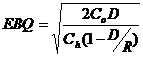(Given in the exams)

Q = the amount produced in each batch

D = demand per annum

Ch = cost of holding one unit for one year

Co = cost of setting up a batch ready to be produced

R = the production rate per time period (which must exceed the inventory usage)

6.     Total Wages

Total wages = (hours worked × basic rate of pay per hour) + (overtime hours worked × overtime premium per hour)

7.     Labor Turnover

No. of leavers who require replacement

Labor turnover = ---------------------------------------------- ----------------- X 100%

Average No. of employees

8. Labor Efficiency

Expected hours to produce actual output (standard hours)

Efficiency ratio = -------------------------------------------------------------------------------- × 100%

Actual hours to produce output

No. of hours spent working (active production)

Capacity ratio = --------------------------------------------------------------------------× 100%

Total hours available (budgeted)

Expected hours to produce actual output (standard hours)

Production volume ratio= -----------------------------------------------------------------------------× 100%

Total hours available (budgeted)

9.     Absorption Rate

Absorption rate = -------------------------------------

Volume of activity

Budgeted production activity

10.     Marginal production cost

Marginal production cost = direct materials + direct labor + variable production overhead

Contribution = Sales – Variable cost

10. Marginal costing VS Absorption costing

If stock levels are rising, AC profit > MC profit

If stock levels are falling, AC profit < MC profit

If opening and closing stock levels are the same, AC profit = MC profit

Difference in profit = Change in inventory level × Fixed overheads absorption per unit

11. Conversion cost

Conversion cost = direct labor + production overheads

12. Correlation Coefficient

 (Given in the exams)Where x and y represent pairs of data for two variables x and yn = the number of pairs of data used in the analysis

Interpretation：

R = +1 perfect positive linear correlation

R between 0 and +1, positive correlation

R=0 no correlation

R between 0 and -1, negative correlation

R = -1 perfect negative linear correlation

13. Interest

l Simple interest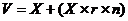Where: V =Future value

X =Initial investment (present value)

r = Interest rate (expressed as a decimal)

n = Number of time periods

l Compound interest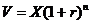Where: V =Future value

X =Initial investment (present value)

r = Interest rate (expressed as a decimal)

n = Number of time periods

l Effective interest rate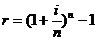Where: r = Effective interest rate

i = Nominal interest rate

n = Number of time periods

14. IRR (using linear interpolation)

The steps in linear interpolation are:

(1)   Calculate two NPVs for the project at two different costs of capital

(2)   Using the following formula to find the IRR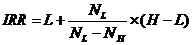Where: L = Lower rate of interest

H = Higher rate of interest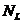= NPV at lower rate of interest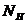= NPV at higher rate of interest

15. Payback Period

Payback period =16. Annuity

PV = Annual cash flow X Annuity Factor

Annuity Factor: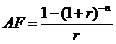17. Perpetuity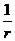is known as the perpetuity factor

IRR of a perpetuity =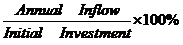18. Variance

l Material price variance = Actual materials bought       should cost－Did cost

l Material usage variance = (Actual output produced should use－Did use) × Standard price

l Labor rate variance = Actual hours worked should cost－Did cost

l Labor efficiency variance = (Actual output produced should take－Did take)   ×Standard rate

l Variable overhead expenditure variance = Actual hours worked should cost－Did cost

l Variable overhead efficiency variance = (Actual output produced should take－Did take)× standard rate

l Fixed overhead absorption rate = budgeted fixed overhead / budgeted activity level

l Fixed overhead expenditure variance = Budget expenditure － Actual expenditure

l Fixed overhead volume variance = (Budget production volume in standard hours － Actual production volume in standard hours) × standard fixed overhead rate

l Fixed overhead volume Capacity variance = (actual hrs worked – budgeted hrs) × absorption rate

l Fixed overhead volume Efficiency variance = (standard hrs worked for actual production － actual hrs worked) × absorption rate

l Sales price variances = Actual sales units × ( Budget price － Actual price)

l Sales volume variances

(Budget sales units － Actual sales units) × standard profit per unit (Absorption costing)

Or × standard contribution per unit (Marginal costing)

19. Profitability measures

l Gross profit margin = Gross profit /Turnover

l Net profit margin= Net profit /Turnover

l ROCE=Profit/capital employed

*Capital employed =total assets – current liabilities

=total equity plus long-term debt

Profit =profit before interest and tax (PBIT)

l Asset turnover= sales × capital employed.

l ROCE =net profit margin ×asset turnoverl Residual Income

RI=Controllable profit —Notional interest on capital

Notional interest on capital = the capital employed X a notional cost of capital or interest rate.

20. Liquidity measures

l Current ratio= current assets/ current liability

l Quick ratio = (current assets-inventory) / current liability

l Receivable collection period =trade receivables ÷ sales × 365 days

l Inventory turnover period=inventory ÷ cost of sales × 365 days

l Inventory turnover=Cost of sales ÷ inventory

l Account payable payment period = (payables/purchases) ×365 days

l Working capital period= (working capital/cost of sales) ×365 days

or = (working capital/ operating costs) ×365 days

l21. Cost per unit of process outputs

 Process cost = \$( cost incurred - scrap value of normal loss - NRV of by-product                  + disposal cost of normal loss) Expected output Input - normal loss

22. Concept of equivalent units

The FIFO method

Cost incurred in current period

Cost per EU = _______________________________________________________________

Opening WIP *(1- 上期完工率) + 本期开工且完工产品 + Closing WIP*本期完工率

Weighted average method

Cost of opening WIP in last period + Cost incurred in current period

Cost per EU = _______________________________________________________________

Opening WIP + 本期开工且完工产品 + Closing WIP*本期完工率

——————————————

## 报考指南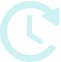距下次考试还有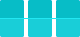天 考试安排 注意事项 考点查询 冲刺串讲 备考建议 成绩公布

## 通关方案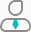## 专家团队带队精讲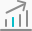## 从零开始分段学习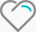## 免费资料## 加入通关小分队APP下载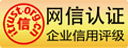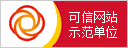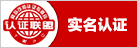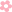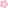24小时购课大优惠哟！